# Python | Pandas Series.combine()

Pandas series is a One-dimensional ndarray with axis labels. The labels need not be unique but must be a hashable type. The object supports both integer- and label-based indexing and provides a host of methods for performing operations involving the index.

Pandas` Series.combine()` function combine the Series with a Series or scalar according to func. It combine the Series and other using func to perform element-wise selection for combined Series. fill_value is assumed when value is missing at some index from one of the two objects being combined.

Syntax: Series.combine(other, func, fill_value=None)

Parameter :
other : Series or scalar
func : Function that takes two scalars as inputs and returns an element.
fill_value : The value to assume when an index is missing from one Series or the other.

Returns : Series

Example #1: Use `Series.combine()` function to find the maximum value for each index labels in the two series object.

 `# importing pandas as pd ` `import` `pandas as pd ` ` `  `# Creating the first Series ` `sr1 ``=` `pd.Series([``80``, ``25``, ``3``, ``25``, ``24``, ``6``]) ` ` `  `# Creating the second Series ` `sr2 ``=` `pd.Series([``34``, ``5``, ``13``, ``32``, ``4``, ``15``]) ` ` `  `# Create the Index ` `index_ ``=` `[``'Coca Cola'``, ``'Sprite'``, ``'Coke'``, ``'Fanta'``, ``'Dew'``, ``'ThumbsUp'``] ` ` `  `# set the first index ` `sr1.index ``=` `index_ ` ` `  `# set the second index ` `sr2.index ``=` `index_ ` ` `  `# Print the first series ` `print``(sr1) ` ` `  `# Print the second series ` `print``(sr2) `

Output :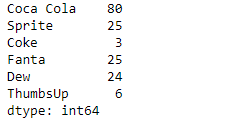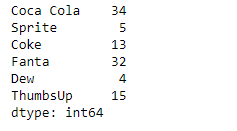Now we will use `Series.combine()` function to find the maximum value for each index labels in the two given series object.

 `# find the maximum element-wise ` `# among sr1 and sr2 ` `result ``=` `sr1.combine(other ``=` `sr2, func ``=` `max``) ` ` `  `# Print the result ` `print``(result) `

Output :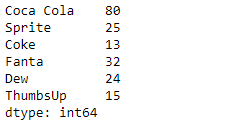As we can see in the output, the `Series.combine()` function has successfully returned the maximum value for each index labels among the two series objects.

Example #2 : Use `Series.combine()` function to find the minimum value for each index labels in the two series object.

 `# importing pandas as pd ` `import` `pandas as pd ` ` `  `# Creating the first Series ` `sr1 ``=` `pd.Series([``51``, ``10``, ``24``, ``18``, ``None``, ``84``, ``12``, ``10``, ``5``, ``24``, ``2``]) ` ` `  `# Creating the second Series ` `sr2 ``=` `pd.Series([``11``, ``21``, ``8``, ``18``, ``65``, ``18``, ``32``, ``10``, ``5``, ``32``, ``None``]) ` ` `  `# Create the Index ` `index_ ``=` `pd.date_range(``'2010-10-09'``, periods ``=` `11``, freq ``=``'M'``) ` ` `  `# set the first index ` `sr1.index ``=` `index_ ` ` `  `# set the second index ` `sr2.index ``=` `index_ ` ` `  `# Print the first series ` `print``(sr1) ` ` `  `# Print the second series ` `print``(sr2) `

Output :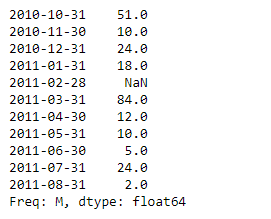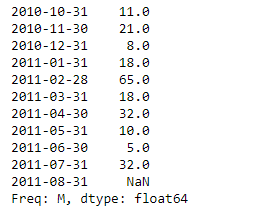Now we will use `Series.combine()` function to find the minimum value for each index labels in the two given series object.

 `# find the minimum element-wise ` `# among sr1 and sr2 ` `result ``=` `sr1.combine(other ``=` `sr2, func ``=` `min``) ` ` `  `# Print the result ` `print``(result) `

Output :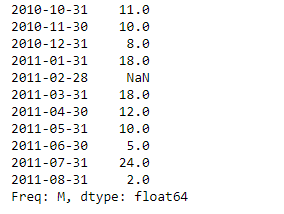As we can see in the output, the `Series.combine()` function has successfully returned the minimum value for each index labels among the two series objects.

My Personal Notes arrow_drop_upCheck out this Author's contributed articles.

If you like GeeksforGeeks and would like to contribute, you can also write an article using contribute.geeksforgeeks.org or mail your article to contribute@geeksforgeeks.org. See your article appearing on the GeeksforGeeks main page and help other Geeks.

Please Improve this article if you find anything incorrect by clicking on the "Improve Article" button below.

Article Tags :

Be the First to upvote.

Please write to us at contribute@geeksforgeeks.org to report any issue with the above content.